Examples

Chapter 7 Class 11 Permutations and Combinations (Term 2)
Serial order wise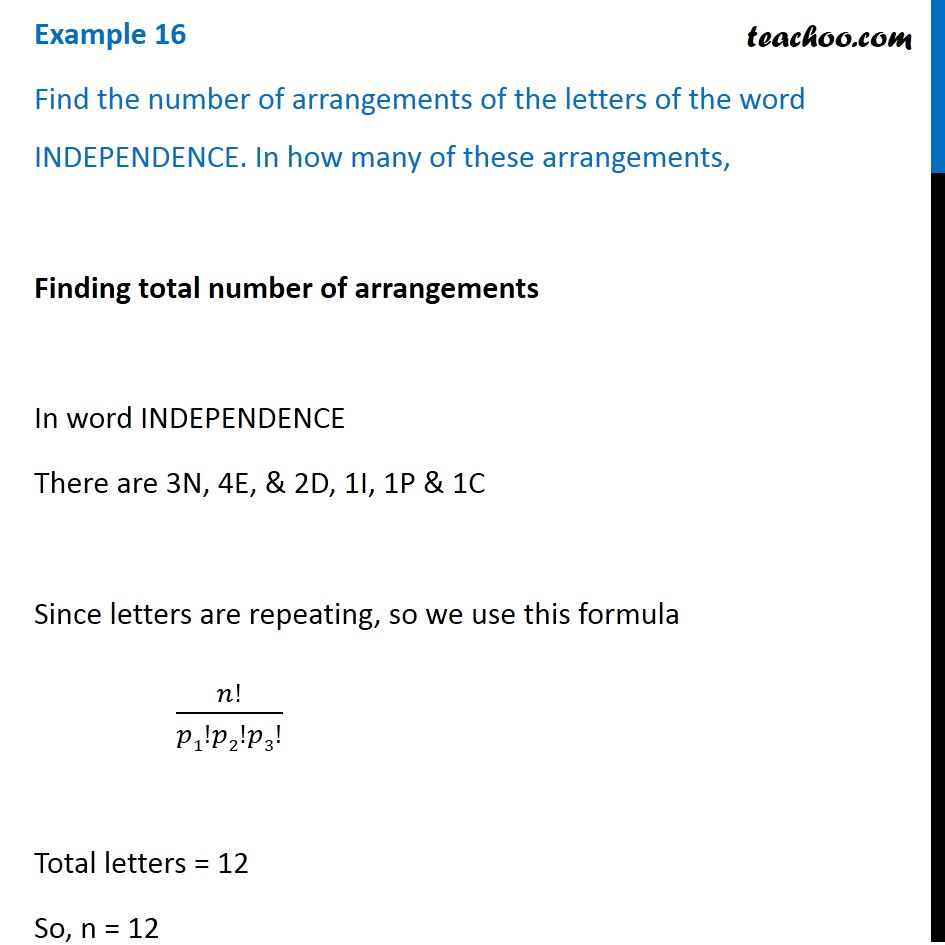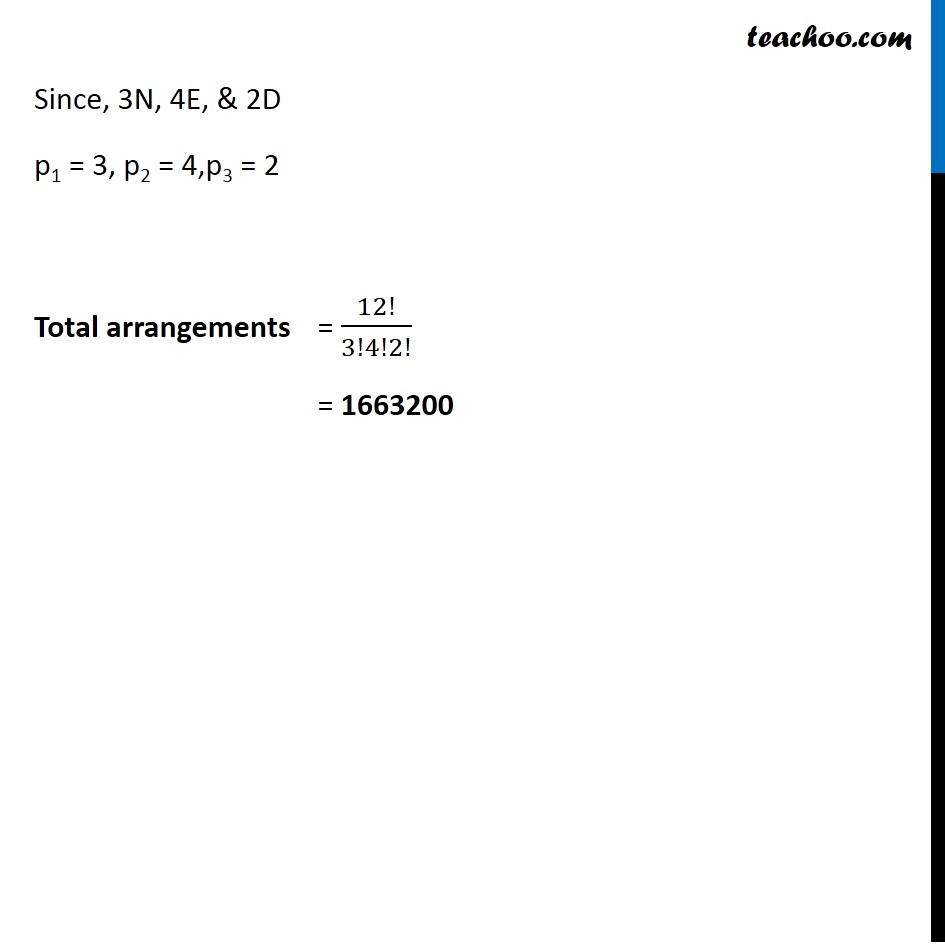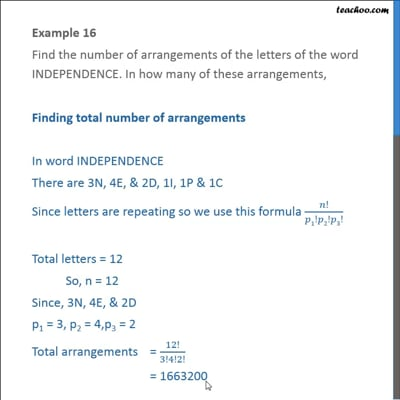This video is only available for Teachoo black users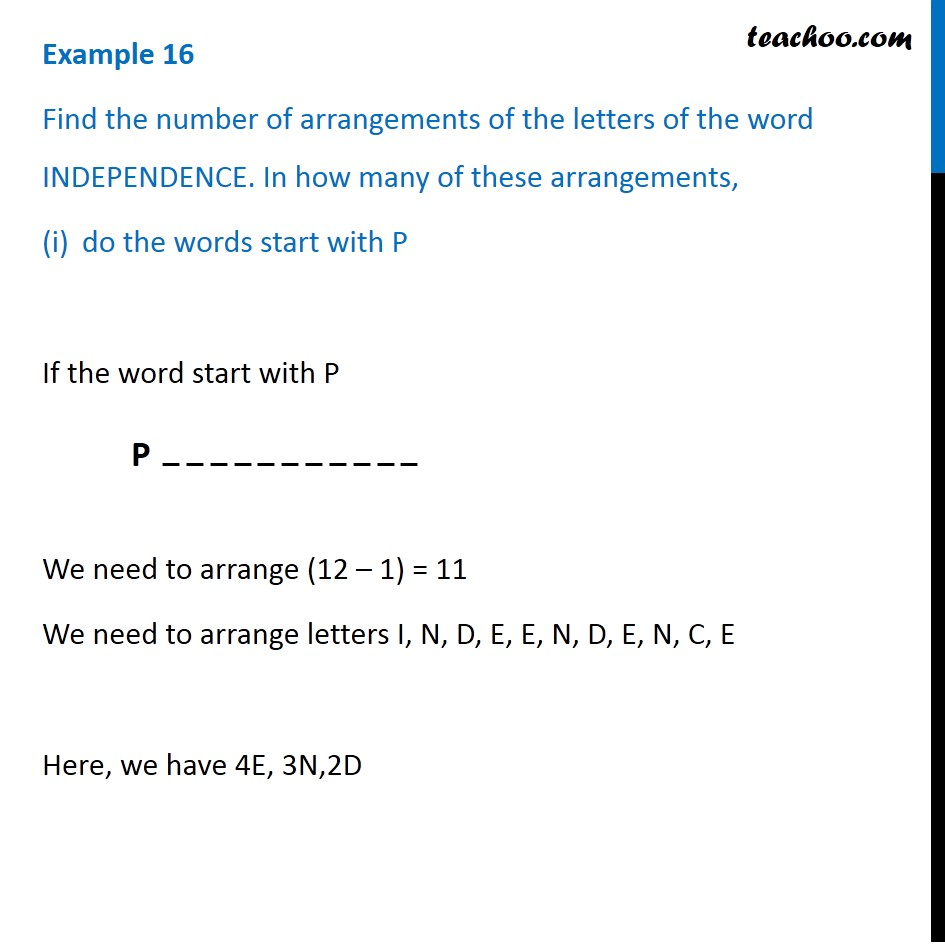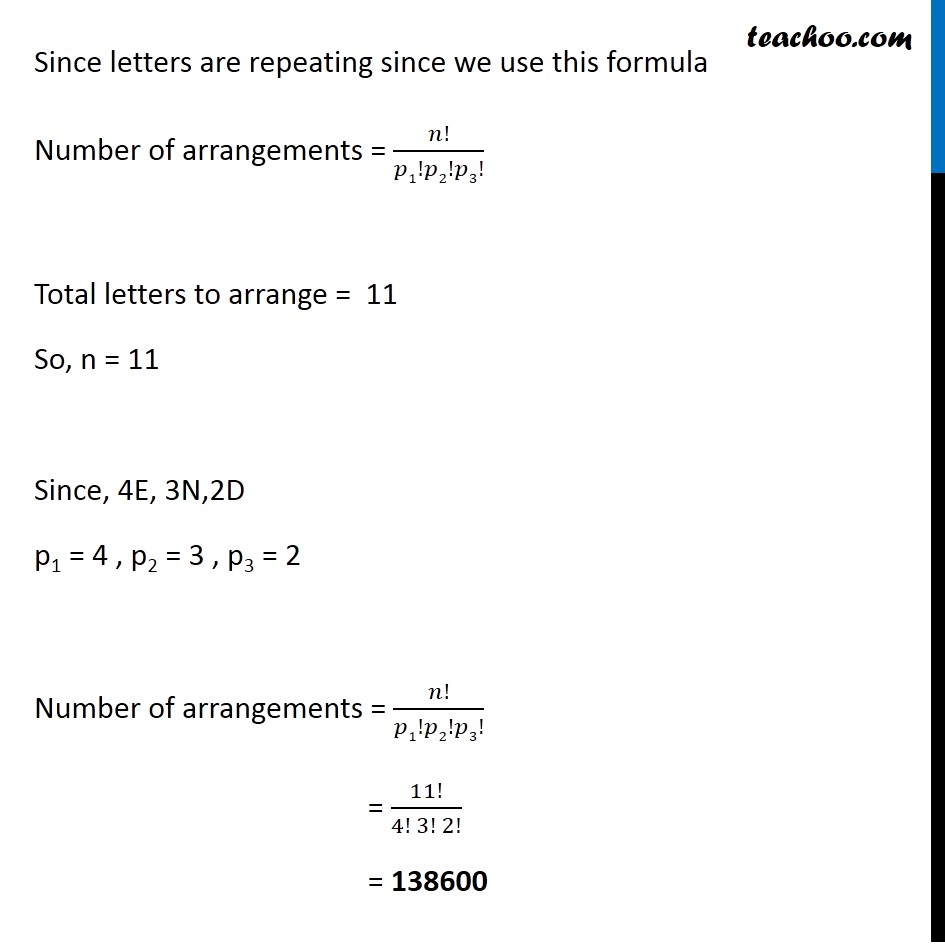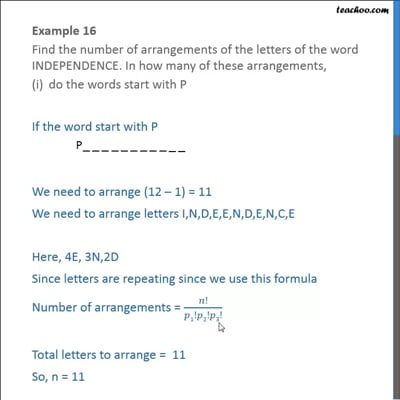This video is only available for Teachoo black users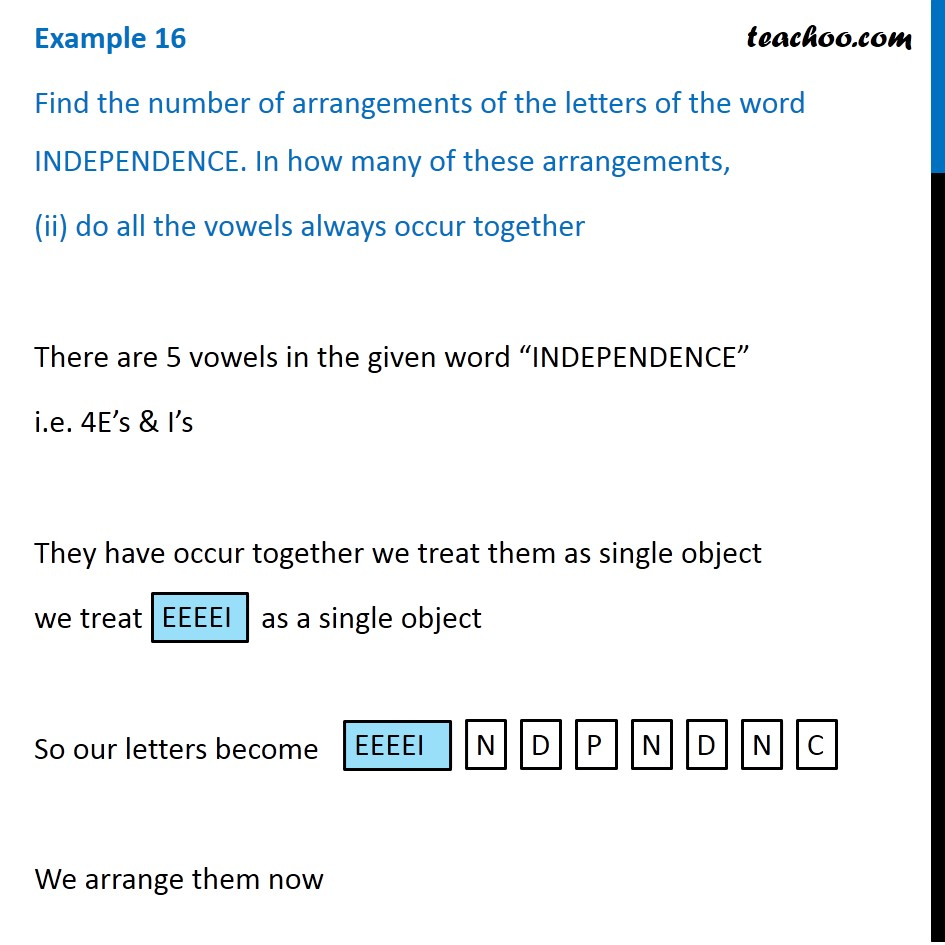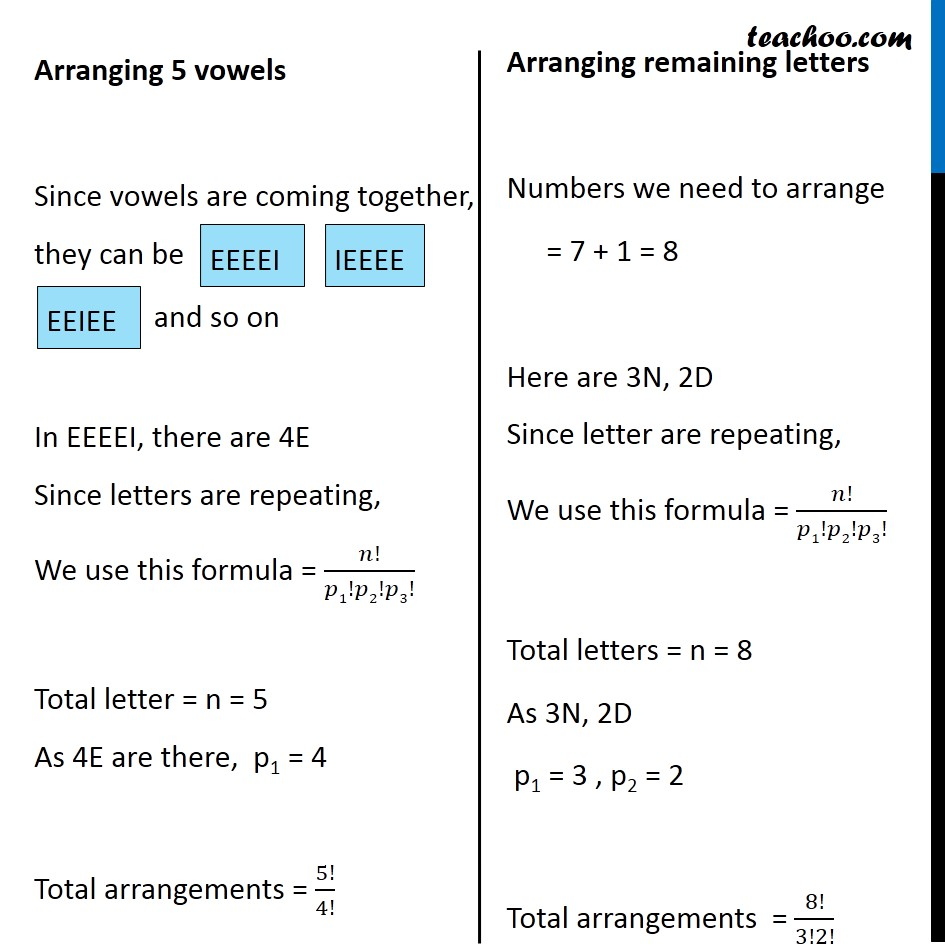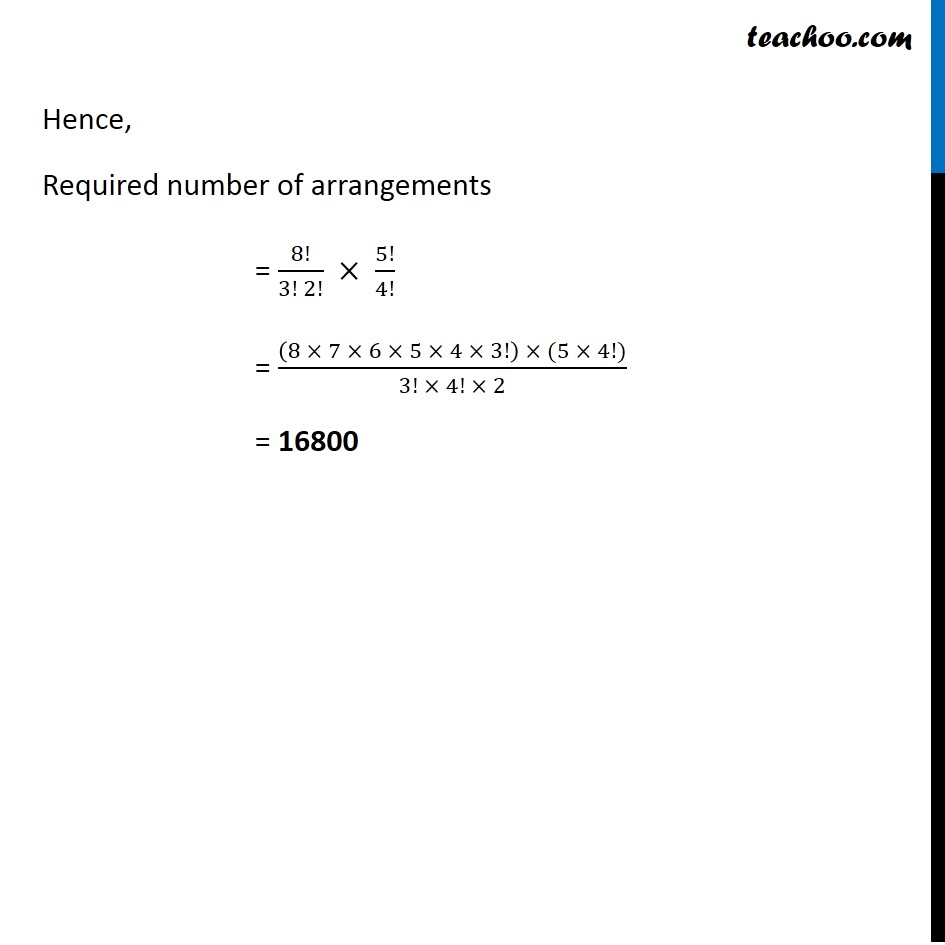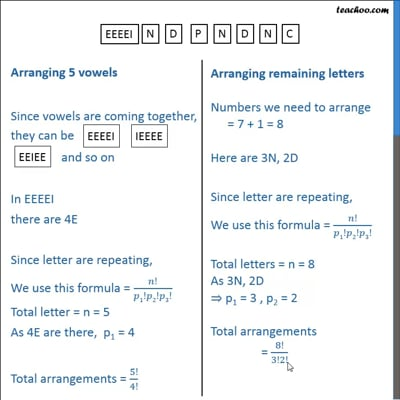This video is only available for Teachoo black users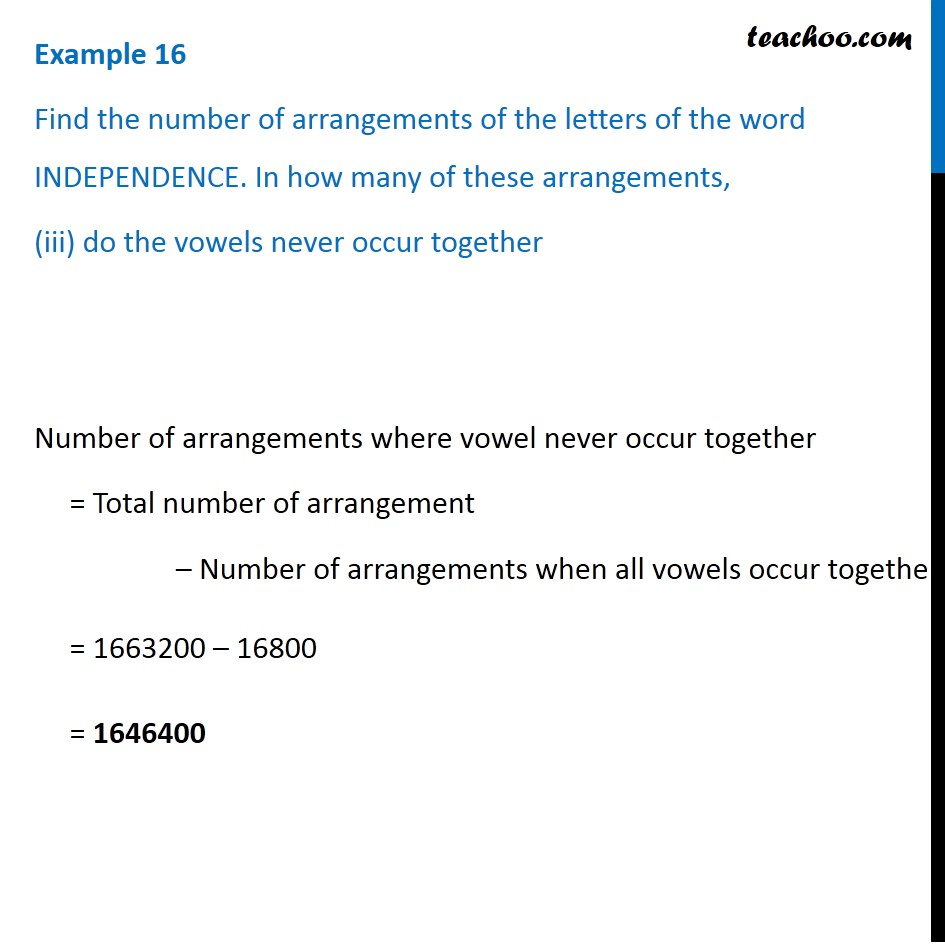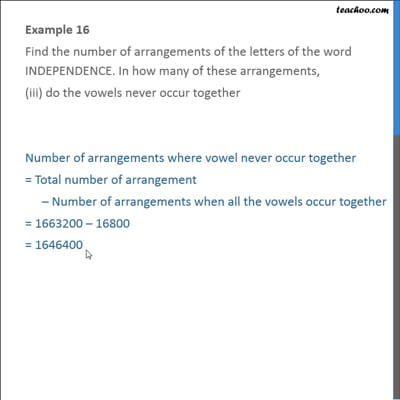This video is only available for Teachoo black users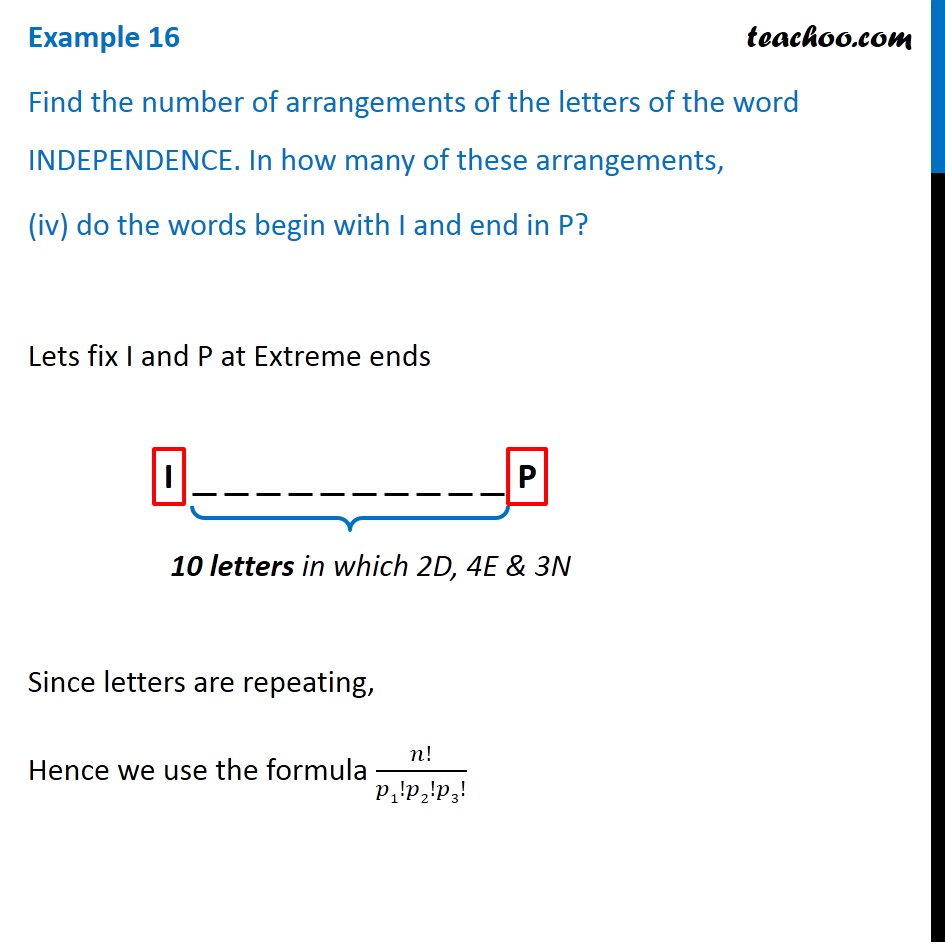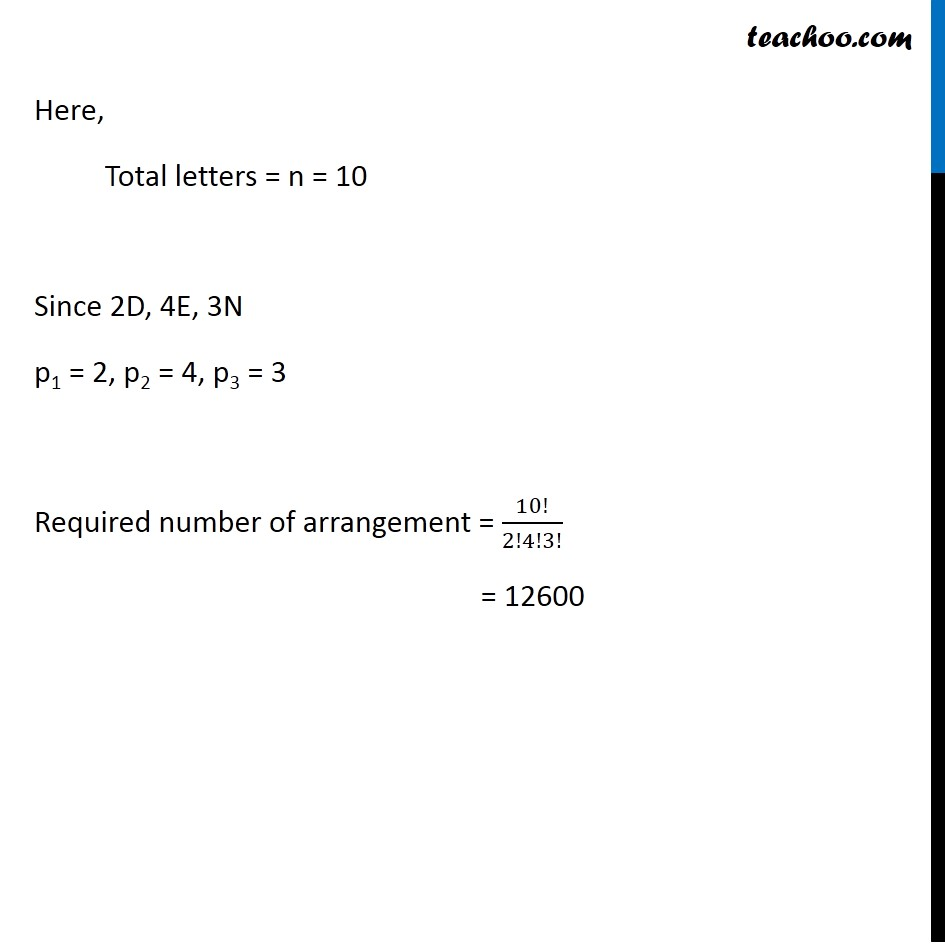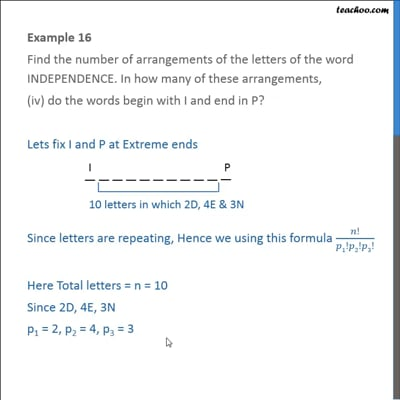This video is only available for Teachoo black users

### Transcript

Example 16 Find the number of arrangements of the letters of the word INDEPENDENCE. In how many of these arrangements, Finding total number of arrangements In word INDEPENDENCE There are 3N, 4E, & 2D, 1I, 1P & 1C Since letters are repeating, so we use this formula 𝑛!/𝑝1!𝑝2!𝑝3! Total letters = 12 So, n = 12 Since, 3N, 4E, & 2D p1 = 3, p2 = 4,p3 = 2 Total arrangements = 12!/3!4!2! = 1663200 Example 16 Find the number of arrangements of the letters of the word INDEPENDENCE. In how many of these arrangements, do the words start with P If the word start with P We need to arrange (12 – 1) = 11 We need to arrange letters I, N, D, E, E, N, D, E, N, C, E Here, we have 4E, 3N,2D Since letters are repeating since we use this formula Number of arrangements = 𝑛!/𝑝1!𝑝2!𝑝3! Total letters to arrange = 11 So, n = 11 Since, 4E, 3N,2D p1 = 4 , p2 = 3 , p3 = 2 Number of arrangements = 𝑛!/𝑝1!𝑝2!𝑝3! = 11!/(4! 3! 2!) = 138600 Example 16 Find the number of arrangements of the letters of the word INDEPENDENCE. In how many of these arrangements, (ii) do all the vowels always occur together There are 5 vowels in the given word “INDEPENDENCE” i.e. 4E’s & I’s They have occur together we treat them as single object we treat as a single object So our letters become We arrange them now Arranging 5 vowels Since vowels are coming together, they can be and so on In EEEEI, there are 4E Since letters are repeating, We use this formula = 𝑛!/𝑝1!𝑝2!𝑝3! Total letter = n = 5 As 4E are there, p1 = 4 Total arrangements = 5!/4! Arranging remaining letters Numbers we need to arrange = 7 + 1 = 8 Here are 3N, 2D Since letter are repeating, We use this formula = 𝑛!/𝑝1!𝑝2!𝑝3! Total letters = n = 8 As 3N, 2D p1 = 3 , p2 = 2 Total arrangements = 8!/3!2! Hence, Required number of arrangements = 8!/(3! 2!) × 5!/4! = ((8 × 7 × 6 × 5 × 4 × 3!) × (5 × 4!))/(3! × 4! × 2) = 16800 Example 16 Find the number of arrangements of the letters of the word INDEPENDENCE. In how many of these arrangements, (iii) do the vowels never occur together Number of arrangements where vowel never occur together = Total number of arrangement – Number of arrangements when all vowels occur together = 1663200 – 16800 = 1646400 Example 16 Find the number of arrangements of the letters of the word INDEPENDENCE. In how many of these arrangements, (iv) do the words begin with I and end in P? Lets fix I and P at Extreme ends Since letters are repeating, Hence we use the formula 𝑛!/𝑝1!𝑝2!𝑝3! Here, Total letters = n = 10 Since 2D, 4E, 3N p1 = 2, p2 = 4, p3 = 3 Required number of arrangement = 10!/2!4!3! = 12600